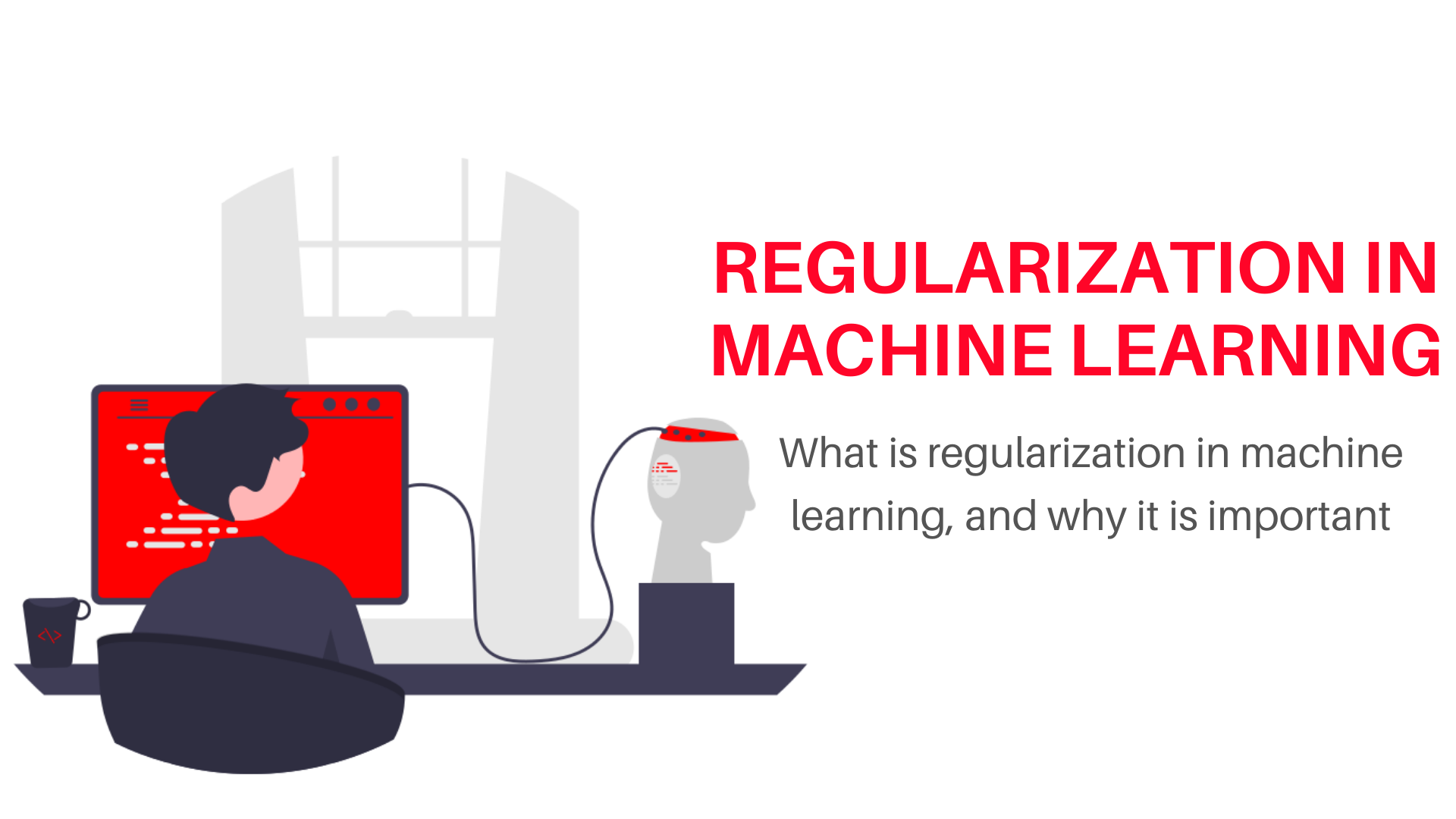# Regularization in machine learning: why is it better 2022?## Introduction

Regularization in machine learning is an important technique to avoid overfitting. Most of the time our machine learning models suffer from overfitting. Overfitting is a phenomenon where the test accuracy is a lot less than the training accuracy. This is due to the model getting overly trained or sometimes it is due to the presence of noise in the data that our model captures. Due to these reasons, our model performs poorly on the unseen data.

In such cases how do we increase the performance of our model? There are many techniques like increasing training data, cross-validation, more feature engineering. But these techniques also have some limitations. The most important technique that works almost all times is Regularization. There are different kinds of regularization techniques. In this article, we will discuss Regularization and its importance in Machine Learning.

## Overview

• What is Regularization?
• Types of Regularization
• Why Regularization is needed?
• How does Regularization work?
• Conclusion

## What is Regularization?

Regularization refers to a technique that reduces the overfitting of a model by penalizing the co-efficient estimates so that model doesn’t learn the noise in the data and hence avoids overfitting or underfitting. Using these techniques we can avoid errors and we can increase the performance of our model.

In the above image, we can see how the performance of the model increased after regularization. The curve became more smooth when compared with the previous standard fit. Here regularization technique helped us to reduce the magnitude of the features by keeping the same number of features. In this way, the regularization technique helps us to deal with high dimensionality problems too.

## Types of Regularization

There are three types of Regularization that are used most commonly. They are as follows

• L1 Regularization
• L2 Regularization
• Drop Out Regularization

L1 Regularization is also known as Lasso regularization and works by penalizing the cost function with the sum of the absolute value of coefficients in the cost function. Similarly, L2 Regularization, also known as Ridge Regularization works by penalizing the cost function with the sum of the squares of the magnitude of coefficients in the cost function. The third type of regularization technique is Drop out regularization which is used in neural networks and this works by randomly ignoring the layers during training thereby preventing complex co-adaptations on training data. The below figure shows how these regularization techniques work overtime.

Let’s see a comparison of these three regularization techniques

## Why Regularization is needed?

Most of the time, when we build a model, our model may not perform well on the unseen data or say test data. Our model either underfits or overfits the data. When we see a very low test accuracy and high training accuracy then we call it overfitting. When we see both test and train accuracies low then it is a condition of underfitting.

We can clearly see, in the above illustration, how underfitting and overfitting occur. This is due to noise in the data that our model learns. Regularization penalizes the hyperparameter that controls the overfitting, underfitting and gets a smooth curve that generalizes our data. So let’s see how regularization works.

## How does regularization work?

The regularization technique works by penalizing the learning parameters. It reduces the error and hence overfitting and underfitting by decreasing the regularization parameters as seen in the below illustration –

We can clearly see in the above illustration that the curve gets smoother when regularization is penalized and the curve gets rough when the parameter lambda is increased. So this is why the regularization technique is used in machine learning.

In the above illustrations, we can see how these regularizations change over a period of time by penalizing the parameters. This is how regularization techniques work.

## Conclusion

In this article, we have discussed different types of regularization techniques and their way of working. Regularization techniques work to reduce overfitting and underfitting in the machine learning and deep learning models. It is better to use regularization in our models to increase the performance of the models and to reduce errors. This is why the Regularization technique is better and is very important while building a machine learning model.##### Aman Kumar

Data Scientist with 3+ years of experience in building data-intensive applications in diverse industries. Proficient in predictive modeling, computer vision, natural language processing, data visualization etc. Aside from being a data scientist, I am also a blogger and photographer.